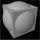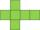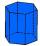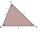Solid geometry, stereometry - page 27

Solid geometry is the name for the geometry of three-dimensional Euclidean space.

Stereometry deals with the measurements of volumes of various solid figures (three-dimensional figures) including pyramids, prisms and other polyhedrons; cylinders; cones; truncated cones; and balls bounded by spheres.

1. Hexa prismDetermine the volume of hex prism with edge base 4 cm. The body height is 28 cm.
2. Square vs rectangleSquare and rectangle have the same area contents. The length of the rectangle is 9 greater and width 6 less than side of the square. Calculate the side of a square.
3. PrismCalculate the surface area and volume of a prism with a body height h = 10 cm and its base has shape of a rhomboid with sides a = 5.8 cm, b = 3 cm and the distance of its two longer sides is w = 2.4 cm.
4. Surface and volume od cuboidContent area of the square base of cuboid is Sp = 36 cm2 and its height 80 mm. Determine its surface area and volume.
5. Cube from sphereWhat largest surface area (in cm2) can have a cube that was cut out of a sphere with radius 43 cm?
6. Rotating coneCalculate the volume and the surface area of a rotating cone of base radius r = 2.3 dm and a height h = 46 mm.
7. The potDiameter of the pot 38 cm. The height is 30 cm. How many liters of water can fit in the pot?
8. Rectangle poolDetermine dimensions of open pool with a square bottom with a capacity 32 m3 to have painted/bricked walls with least amount of material.
9. FloorRectangular floor of living room has a length 5.4 meters and a circumference 17.2 meters. What is its width?
10. Hexagon rotationA regular hexagon of side 6 cm is rotated through 60° along a line passing through its longest diagonal. What is the volume of the figure thus generated?
11. PaintingTo paint the pool with dimensions: 2 meters depth, 3m x 4m we bought paint to 50 meters square. How many "paint" will be a waste?
12. PrismThe base of a perpendicular triangular prism is a right triangle with legs 4.5 cm and 6 cm long. What is the surface of the prism, if its volume is 54 cubic centimeters?The regular quadrangular prism has a base edge a = 7.1 cm and side edge = 18.2 cm long. Calculate its volume and surface area.
14. Grid/network of cubeGrid of cube has a circumference 42 mm. Calculate the surface area of the cube ...
15. Regular triangular prismCalculate the surface area of body of regular triangular prism, when the length of its base edge is 6.5 cm and height 0.2 m.
16. Cube 5The content area of one cube wall is 32 square centimeters. Determine the length of its edges, its surface and volume.
17. The tentThe tent shape of a regular quadrilateral pyramid has a base edge length a = 2 m and a height v = 1.8 m. How many m2 of cloth we need to make the tent if we have to add 7% of the seams? How many m3 of air will be in the tent?
18. OfficeOffice building was built in the shape of a regular hexagon inscribed in a circle with a radius of 12 m. The height of the walls is 7m. How much CZK cost plastering the walls of the building, if per 1 m square cost CZK 400?
19. Triangular prismCalculate the surface of a triangular prism 10 cm high, the base of which is a triangle with sides 6 cm 8 cm and 8 cm
20. Body diagonalCuboid with base 7cm x 3,9cm and body diagonal 9cm long. Find the height of the cuboid and the length of the diagonal of the base,

Do you have an interesting mathematical example that you can't solve it? Enter it, and we can try to solve it.

To this e-mail address, we will reply solution; solved examples are also published here. Please enter e-mail correctly and check whether you don't have a full mailbox.